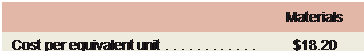### Create an Account

Home / Questions / Krollon Company uses the FIFO method in its process costing system The following data are ...

# Krollon Company uses the FIFO method in its process costing system The following data are for the most recent month of operations in one of the company’s processing departments

Krollon Company uses the FIFO method in its process costing system. The following data are for the most recent month of operations in one of the company’s processing departments:

 Units in beginning inventory . . . . . . . . . . . . . . . . . . . . . . 400 Units started into production . . . . . . . . . . . . . . . . . . . . . . 4,300 Units in ending inventory . . . . . . . . . . . . . . . . . . . . . . . . 300 Units transferred to the next department . . . . . . . . . . . . 4,400 Materials Conversion Percentage completion of beginning inventory . . . . . . . . 70% 30% Percentage completion of ending inventory . . . . . . . . . . 80% 40%

According to the company’s costing system, the cost of beginning inventory was \$7,886, of which

\$4,897 was for materials and the remainder was for conversion cost. The costs added during the month amounted to \$181,652. The costs per equivalent unit for the month were:Required:

1.       Compute the total cost per equivalent unit for the month.

2.       Compute the equivalent units of material and of conversion costs in the ending inventory.

3.       Compute the equivalent units of material and of conversion costs that were required to com- plete the beginning inventory.

4.       Determine the number of units started and completed during the month.

5.       Determine the costs of ending inventory and units transferred out.

Jul 01 2020 View more View LessSubscribe To Get Solution